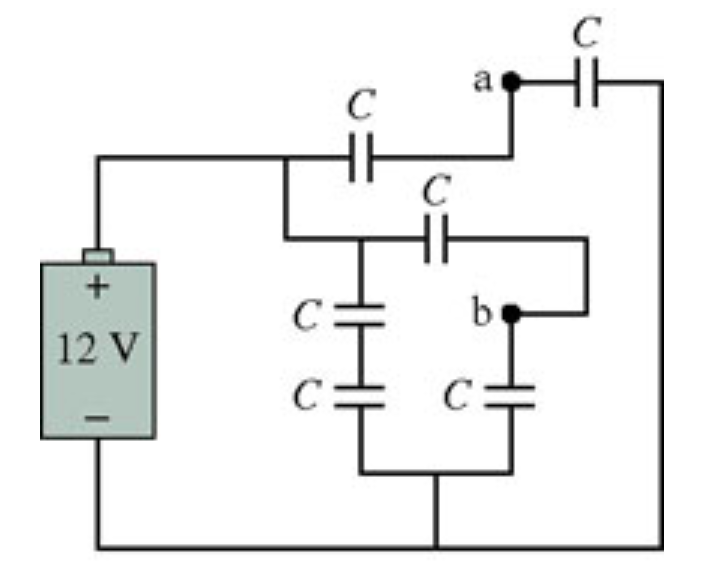# Problem: Six identical capacitors with capacitance C are connected as shown in the figure (Figure 1) What is the potential difference between points a and b?

###### FREE Expert Solution

We'll redraw the circuit for clarity:

85% (187 ratings)###### Problem Details

Six identical capacitors with capacitance C are connected as shown in the figure (Figure 1)What is the potential difference between points a and b?